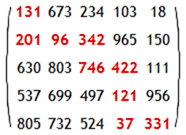# Project Euler – Problem 81 Solution

#### Problem

In the 5 by 5 matrix below, the minimal path sum from the top left to the bottom right, by only moving to the right and down, is indicated in bold red and is equal to 2427.Find the minimal path sum, in matrix.txt (right click and ‘Save Link/Target As…’), a 31K text file containing a 80 by 80 matrix, from the top left to the bottom right by only moving right and down.

#### Solution

I took a similar approach to the one I used for problem 18 and iteratively work out what’s the shortest path sum leading up to each of the cells in the matrix.

Considering that a brute force approach will need to walk through the 80 x 80 matrix a total of 92045125813734238026462263037378063990076729140 times…so that’s clearly not an option! So instead, for each cell (x, y) in the matrix, we consider the two possibilities:

1. the path has traversed down from cell (x-1, y)

2. the path has traversed right from cell (x, y-1)

the shortest path sum from top left to (x, y), let’s call it sps(x, y) will be equal to the lesser of sps(x-1, y) + (x, y) and sps(x, y-1) + (x, y). Obviously there are some special cases, such as when x = 0 and when y = 0, as you can see the different handling in the match pattern.